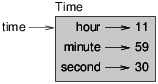# 7.1: Time

$$\newcommand{\vecs}{\overset { \rightharpoonup} {\mathbf{#1}} }$$ $$\newcommand{\vecd}{\overset{-\!-\!\rightharpoonup}{\vphantom{a}\smash {#1}}}$$$$\newcommand{\id}{\mathrm{id}}$$ $$\newcommand{\Span}{\mathrm{span}}$$ $$\newcommand{\kernel}{\mathrm{null}\,}$$ $$\newcommand{\range}{\mathrm{range}\,}$$ $$\newcommand{\RealPart}{\mathrm{Re}}$$ $$\newcommand{\ImaginaryPart}{\mathrm{Im}}$$ $$\newcommand{\Argument}{\mathrm{Arg}}$$ $$\newcommand{\norm}{\| #1 \|}$$ $$\newcommand{\inner}{\langle #1, #2 \rangle}$$ $$\newcommand{\Span}{\mathrm{span}}$$ $$\newcommand{\id}{\mathrm{id}}$$ $$\newcommand{\Span}{\mathrm{span}}$$ $$\newcommand{\kernel}{\mathrm{null}\,}$$ $$\newcommand{\range}{\mathrm{range}\,}$$ $$\newcommand{\RealPart}{\mathrm{Re}}$$ $$\newcommand{\ImaginaryPart}{\mathrm{Im}}$$ $$\newcommand{\Argument}{\mathrm{Arg}}$$ $$\newcommand{\norm}{\| #1 \|}$$ $$\newcommand{\inner}{\langle #1, #2 \rangle}$$ $$\newcommand{\Span}{\mathrm{span}}$$$$\newcommand{\AA}{\unicode[.8,0]{x212B}}$$

As another example of a user-defined type, we’ll define a class called Time that records the time of day. The class definition looks like this:

class Time(object):
"""Represents the time of day.

attributes: hour, minute, second
"""


We can create a new Time object and assign attributes for hours, minutes, and seconds:

time = Time()
time.hour = 11
time.minute = 59
time.second = 30


The state diagram for the Time object looks like Figure 16.1.1.Figure $$\PageIndex{1}$$: Object diagram.

Exercise $$\PageIndex{1}$$

Write a function called print_time that takes a Time object and prints it in the form hour:minute:second. Hint: the format sequence '%.2d' prints an integer using at least two digits, including a leading zero if necessary.

Exercise $$\PageIndex{2}$$

Write a boolean function called is_after that takes two Time objects, t1 and t2, and returns True if t1 follows t2 chronologically and False otherwise. Challenge: don’t use an if statement.

This page titled 7.1: Time is shared under a CC BY-NC-SA 3.0 license and was authored, remixed, and/or curated by Allen B. Downey (Green Tea Press) .